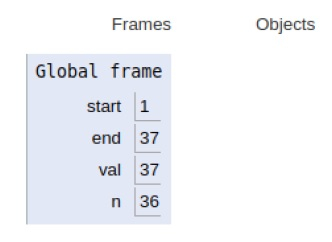# Python program to print all Prime numbers in an Interval

Problem statement − We are given an interval we need to compute all the prime numbers in a given range

Here we will be discussing a brute-force approach to get the solution i.e. the basic definition of a prime number. Prime numbers are the number which has 1 and itself as a factor and rests all the numbers are not its factors.

Each time the condition of a prime number is evaluated to be true computation is performed.

Now let’s observe the concept in the implementation below−

## Example

Live Demo

start = 1
end = 37
for val in range(start, end + 1):
# If num is divisible by any number
# between 2 and val, it is not prime
if val > 1:
for n in range(2, val):
if (val % n) == 0:
break
else:
print(val)

### Output

2
3
5
7
11
13
17
19
23
29
31
37All the variables are declared in the local scope and their references are seen in the figure above.

## Conclusion

In this article, we have learned about how we can print all the prime numbers in an interval